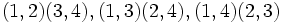# Metabelian group

(diff) ← Older revision | Latest revision (diff) | Newer revision → (diff)

## Definition

QUICK PHRASES: abelian-by-abelian, abelian normal subgroup with abelian quotient, abelian derived subgroup, abelian commutator subgroup

### Symbol-free definition

A group is said to be metabelian or is said to have derived length two or solvable length two if it satisfies the following equivalent conditions:

1. It is solvable of derived length at most two, i.e., the derived series has length at most two.
2. Its derived subgroup (i.e., commutator subgroup with itself) is abelian.
3. There is an abelian normal subgroup that is also an abelian-quotient subgroup, i.e., an abelian normal subgroup with abelian quotient group.
4. Any two commutators (i.e., elements that can be expressed as commutators of elements of the group) commute with each other.
NOTE: Sometimes, the term metabelian or derived length two or solvable length two is used specifically for a group whose derived length is precisely two, i.e., a non-abelian metabelian group. This is more restrictive than the typical usage of the term.

## History

### Origin

The concept and term metabelian group was introduced by Furtwangler in 1930.

The term metabelian was earlier used for groups of nilpotency class two, which is a much stronger condition, but is no longer used in that sense.

## Formalisms

### In terms of the meta operator

This property is obtained by applying the meta operator to the property: abelian group
View other properties obtained by applying the meta operator

The property of being metabelian arises by applying the meta operator to the group property of being Abelian. Equivalently metabelian can be described as Abelian-by-Abelian, where by denotes the group extension operator.

## Examples

VIEW: groups satisfying this property | groups dissatisfying this property
VIEW: Related group property satisfactions | Related group property dissatisfactions

### Generic examples

• The trivial group is metabelian; in fact, it has derived length zero.
• Any abelian group is metabelian; in fact, it has derived length zero.
• Any group of nilpotency class two is metabelian.
• Any group arising as the holomorph of a cyclic group (i.e., the semidirect product of a cyclic group and its automorphism group) is metabelian. This is because cyclic implies abelian automorphism group -- the automorphism group of a cyclic group is abelian. More generally, any group arising as the semidirect product of a cyclic group and any subgroup of its automorphism group is abelian.
• Any dihedral group is metabelian: it has a cyclic normal subgroup and the quotient group is cyclic of order two. (This is a special case of the previous example). It turns out that dihedral groups are nilpotent only if their order is a power of two; further, the nilpotency class of a dihedral group of order$2^n$ is$n - 1$.
• Any generalized dihedral group is metabelian: it has an abelian normal subgroup and the quotient group is cyclic of order two.

### Particular examples

• The symmetric group of degree three, which is also the dihedral group of order six and degree three, is metabelian. It is the smallest example of a metabelian non-abelian group; it is also not nilpotent. The abelian normal subgroup in this case is the alternating group, i.e., the subgroup generated by a$3$-cycle$\langle (1,2,3) \rangle$, and the quotient group is cyclic of order two.
• The dihedral group of order eight (degree four) is metabelian. We have several choices here of the abelian normal subgroup. We can take the center as the abelian normal subgroup, in which case the quotient is isomorphic to a Klein four-group. Alternatively, we could take as our abelian normal subgroup any of the subgroups of order four.
• the alternating group of degree four (order twelve) is metabelian. It has an abelian normal subgroup of order four: the Klein four-subgroup comprising the identity and the three double transpositions$(1,2)(3,4), (1,3)(2,4), (1,4)(2,3)$. The quotient is a cyclic group of order three.

## Relation with other properties

### Stronger properties

Property Meaning Proof of implication Proof of strictness (reverse implication failure) Intermediate notions
cyclic group Abelian group, Group of nilpotency class two, Group whose automorphism group is abelian, Metacyclic group|FULL LIST, MORE INFO
group of nilpotency class two commutator subgroup is central |FULL LIST, MORE INFO
group whose automorphism group is abelian the automorphism group is an abelian group Group of nilpotency class two|FULL LIST, MORE INFO

### Weaker properties

Property Meaning Proof of implication Proof of strictness (reverse implication failure) Intermediate notions
group with nilpotent derived subgroup derived subgroup is nilpotent |FULL LIST, MORE INFO
group satisfying subnormal join property join of subnormal subgroups is subnormal metabelian implies subnormal join property Group with nilpotent derived subgroup|FULL LIST, MORE INFO

## Metaproperties

### Subgroups

This group property is subgroup-closed, viz., any subgroup of a group satisfying the property also satisfies the property
View a complete list of subgroup-closed group properties

Any subgroup of a metabelian group is metabelian. This follows from the general fact that the derived series of the subgroup is contained (entry-wise) in the derived series of the whole group.

### Quotients

This group property is quotient-closed, viz., any quotient of a group satisfying the property also has the property
View a complete list of quotient-closed group properties

Any quotient of a metabelian group is metabelian. This follows from the fact that the derived series of the quotient is the quotient of the derived series of the original group.

### Direct products

This group property is direct product-closed, viz., the direct product of an arbitrary (possibly infinite) family of groups each having the property, also has the property
View other direct product-closed group properties

A direct product of metabelian groups is metabelian. This follows from the fact that the derived series of the direct product is the direct product of the respective derived series.